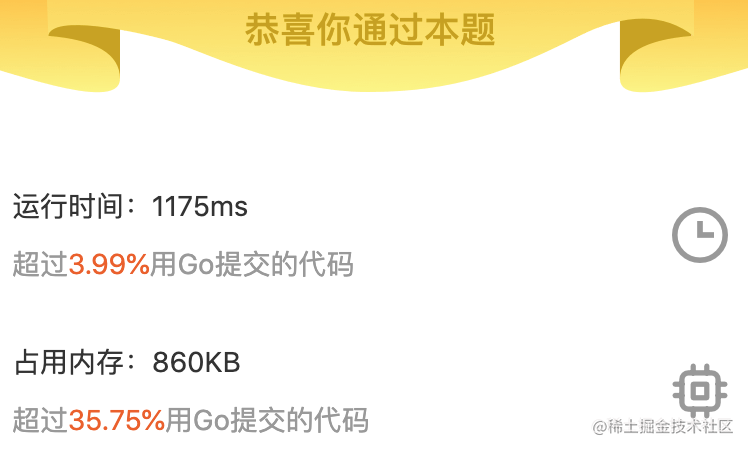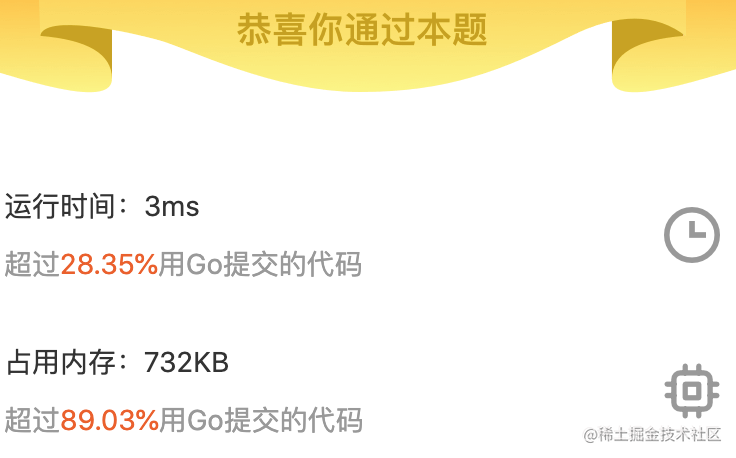# 【剑指Offer 7.斐波那契数列】｜刷题打卡

• 题目描述
• 思路分析
• AC 代码
• 总结

4

3

## 三、AC 代码

``````package main

/**
*
* @param n int整型
* @return int整型
*/
func Fibonacci( n int ) int {
if n == 0 || n == 1 {
return n
}
return Fibonacci(n-1) + Fibonacci(n-2)
}

``````package main

/**
*
* @param n int整型
* @return int整型
*/
func Fibonacci( n int ) int {
a, b := 0, 1
for i := 0; i < n; i++ {
a, b = b, a+b
}
return a
}